# Categorical Data

• Pie Charts• Bar Graphs• Comparative Bar Graphs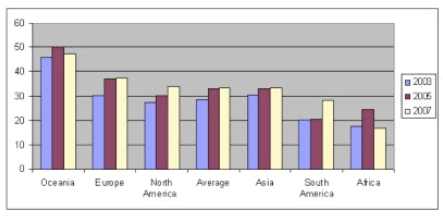• Relative Frequency Bar Graphs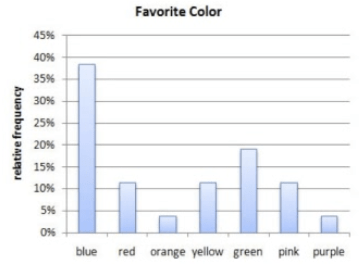# Numerical Data (Discrete)

• Dot Plots• Stem (and leaf) plots# Numerical Data (Continuous)

• Histogram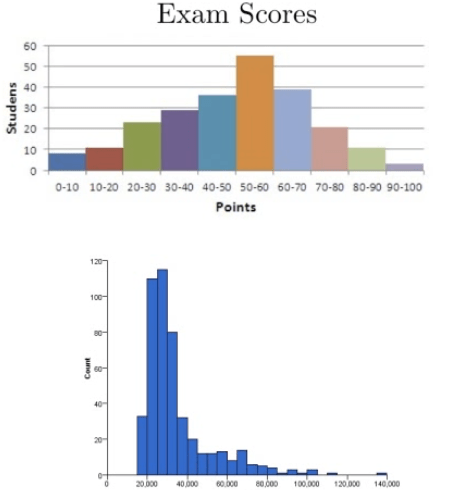# Numerical Data (Cumulative Frequency Plots)

• Frequency Polygon• Ogive Plot# Stem Plots to Compare Two Groups of Data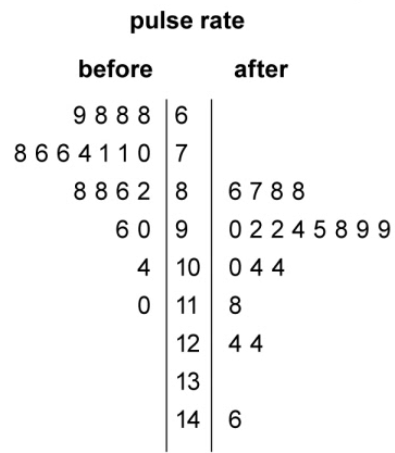• Compare the distribution pulse rate before and after administering a new drug

• After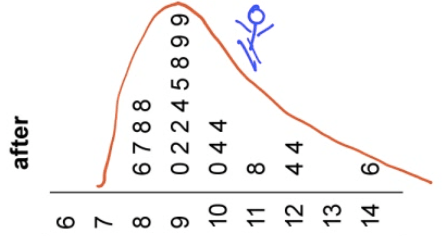• Skewed right

• An outlier at 146

• Centered around 95

• Spread between 86 and 146

• Range of 60

• Before• Skew right

• No outliers

• Lower center at around 70

• Spread between 68 to 110

• Smaller range of 42

# Describe the Distribution

• Shape (only for numerical data)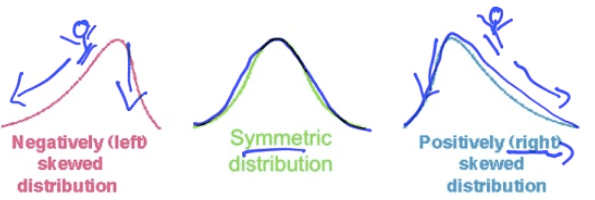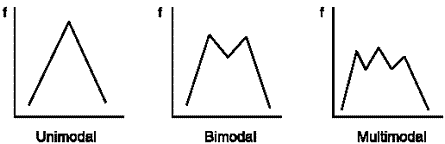# Examples

• Make a stem plot of the ages in a college classroom 18, 18, 17, 21, 26, 40, 23• Use your calculator to make a histogram of ages in a college classroom 18, 18, 17, 21, 26, 40, 23, 27, 22, 19, 20, 21, 18, 35, 32

• STAT ➡️ EDIT ➡️ Enter & Type in the data• STAT PLOT (2ND + Y=) Turn on & Select the type• Zoom + 9• TRACE• Describe the distribution of the graph above

• skew right
• Students took a statistic quiz. The score for the quiz are below. Describe the distribution of the quiz scores• Slightly skewed left

• No outliers

• Centered around 42

• Spread of 8 to 50

• Here are the IQ test scores of a few students. Make a stem plot of these scores.

145 139 126 122 125 130 96 110 120 143• You are interested in how students in your class get to school in the morning. You take a survey and collect the following data:

Car 15, Bus 3, Walk 5, Bicycle 8, Skateboard 1

• Construct a bar graph of your data• Construct a relative frequency bar graph